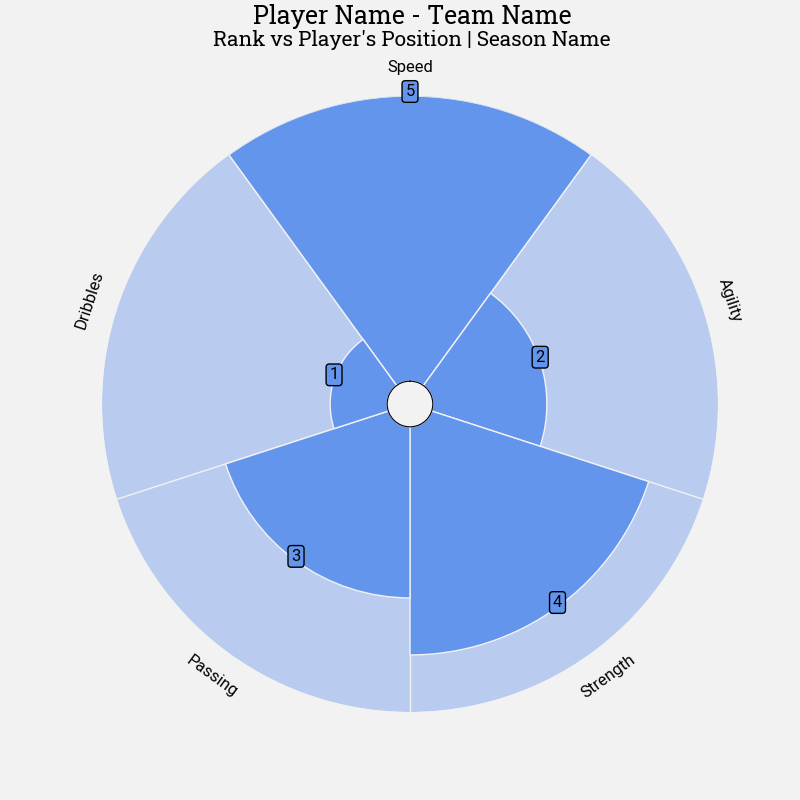# Different Units Pizza

Here we plot a pizza chart where the parameters have the same units, but the maximum is five instead of 100.

```import matplotlib.pyplot as plt

from mplsoccer import PyPizza, FontManager
```

We will use mplsoccer’s FontManager to load some fonts from Google Fonts. We borrowed the FontManager from the excellent ridge_map library.

```font_normal = FontManager('https://raw.githubusercontent.com/google/fonts/main/apache/roboto/'
'Roboto%5Bwdth,wght%5D.ttf')
'Roboto-Italic%5Bwdth,wght%5D.ttf')
'RobotoSlab%5Bwght%5D.ttf')
```

## Different Units

Till now we were plotting a percentile chart where the upper limit was 100. Let’s take another example where the lower limit is 0 and upper limit is 5. The below code shows how to plot pizza-chart for such case.

```# parameter and value list
params = ['Speed', 'Agility', 'Strength', 'Passing', 'Dribbles']
values = [5, 2, 4, 3, 1]

# instantiate PyPizza class
baker = PyPizza(
params=params,                  # list of parameters
straight_line_color="#F2F2F2",  # color for straight lines
straight_line_lw=1,             # linewidth for straight lines
straight_line_limit=5.0,        # max limit of straight lines
last_circle_lw=0,               # linewidth of last circle
other_circle_lw=0,              # linewidth for other circles
inner_circle_size=0.4,          # size of inner circle
)

# plot pizza
fig, ax = baker.make_pizza(
values,                     # list of values
color_blank_space="same",   # use same color to fill blank space
blank_alpha=0.4,            # alpha for blank-space colors
param_location=5.5,         # where the parameters will be added
kwargs_slices=dict(
facecolor="cornflowerblue", edgecolor="#F2F2F2",
zorder=2, linewidth=1
),                          # values to be used when plotting slices
kwargs_params=dict(
color="#000000", fontsize=12,
fontproperties=font_normal.prop, va="center"
),                          # values to be used when adding parameter
kwargs_values=dict(
color="#000000", fontsize=12,
fontproperties=font_normal.prop, zorder=3,
bbox=dict(
edgecolor="#000000", facecolor="cornflowerblue",
)
)                           # values to be used when adding parameter-values
)

fig.text(
0.515, 0.97, "Player Name - Team Name", size=18,
ha="center", fontproperties=font_bold.prop, color="#000000"
)

fig.text(
0.515, 0.942,
"Rank vs Player's Position | Season Name",
size=15,
ha="center", fontproperties=font_bold.prop, color="#000000"
)

plt.show()
```Total running time of the script: ( 0 minutes 0.410 seconds)

Gallery generated by Sphinx-Gallery